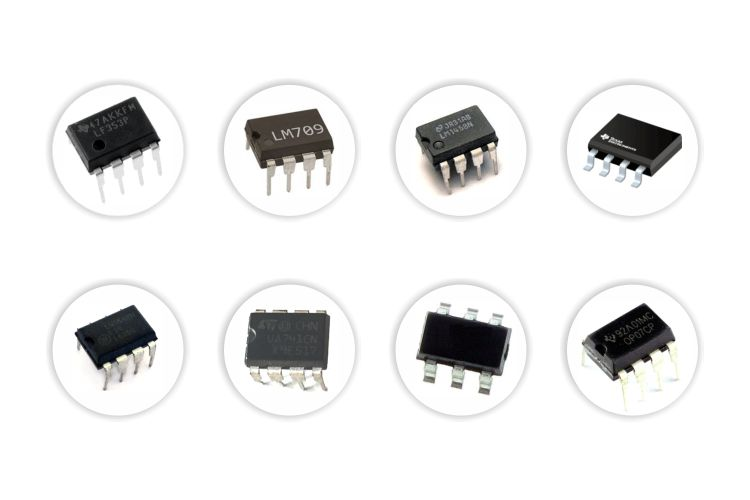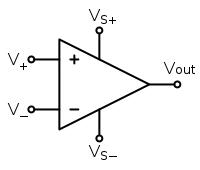## OP Amp

What is an OP amp?Hint
An operational amplifier (often op amp or opamp) is usually depicted in circuit diagram problems as a triangle.An amplifier takes an incoming electronic signal, and outputs a larger version of that same signal. An OP amp (operational amplifier) is a type of amplifier with two inputs (one positive and the other negative), an output, and two power supplies (one a positive voltage, and the other negative). It is usually depicted as a triangle in circuit diagrams.The word Op-amp implies specific properties:
• High gain where ~ $$A=10^5\leftrightarrow 10^6$$
• Used in feedback circuits
• Differential inputs (two wire inputs as opposed to single wire)
• $$v_{out}=Gain\times (v^{+}-v^{-})=A\times v_{in}$$
• The input current is typically zero ( $$i=0)$$

In essence, it is a differential amplifier with really high gain. The Op-amp physically is a complex design typically comprised of ~50 transistors, resistors, and sometimes capacitors. However, knowing that input current = 0, and $$v_{out}=A\times v_{in}$$ , we can solve most circuit problems.

An amplifier takes an incoming electronic signal, and outputs a larger version of that same signal. An OP amp (operational amplifier) is a type of amplifier with two inputs (one positive and the other negative), an output, and two power supplies (one a positive voltage, and the other negative). It is usually depicted as a triangle in circuit diagrams.